why

• 累计撰写 197 篇文章
• 累计创建 11 个标签
• 累计收到 114 条评论

# 构建一个高效且可伸缩的缓存，最后一集。2022-02-24 / 0 评论 / 3 点赞 / 798 阅读 / 5,936 字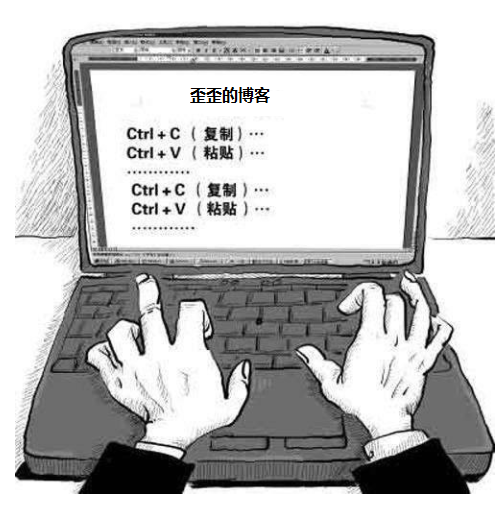public class ScoreQueryService {

public static final Map<String, Future<Integer>> SCORE_CACHE = new ConcurrentHashMap<>();

public Integer query(String userName) throws Exception {
while (true) {
if (future == null) {
//如果为空说明之前这个 key 在 map 里面不存在
if (future == null) {
}
}
try {
return future.get();
} catch (CancellationException e) {
} catch (Exception e) {
throw e;
}
}
}

//模拟耗时
TimeUnit.SECONDS.sleep(5);
}
}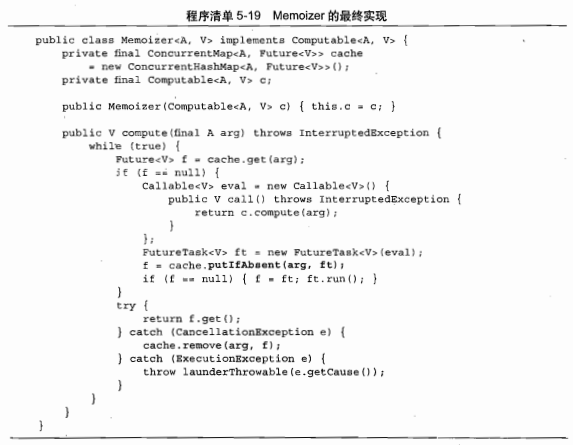## 垃圾代码## 装饰模式

public interface Computable<A, V> {
V compute(A arg) throws Exception;
}


Computable，这个词儿应该认识吧，四级必考词汇，得学起来：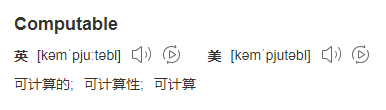public class ScoreQuery implements Computable<String, Integer> {

@Override
public Integer compute(String userName) throws Exception {
//模拟耗时
TimeUnit.SECONDS.sleep(5);
}
}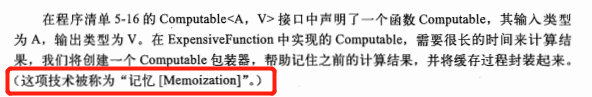public class Memoizer<A, V> implements Computable<A, V> {

@Override
public V compute(A arg) throws Exception {
return null;
}
}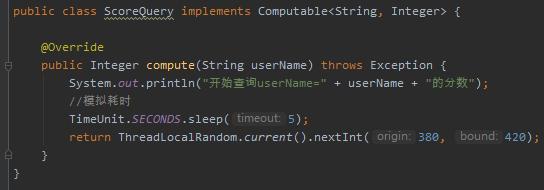public class Memoizer<A, V> implements Computable<A, V> {

private final Computable<A, V> c;

public Memoizer(Computable<A, V> c) {
this.c = c;
}

@Override
public V compute(A arg) throws Exception {
return c.compute(arg);
}
}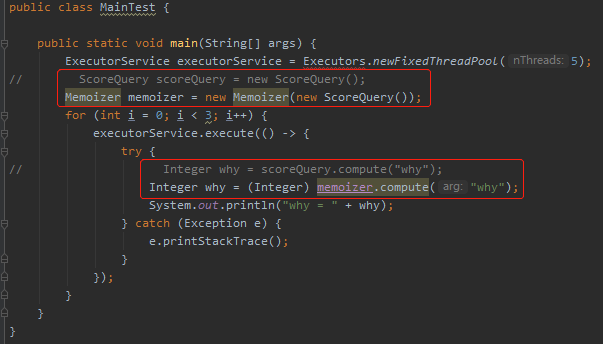Memoizer memoizer = new Memoizer(new ScoreQuery());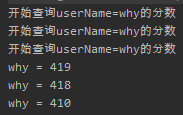public class Memoizer<A, V> implements Computable<A, V> {

private final Map<A, V> cache = new HashMap<A, V>();

private final Computable<A, V> c;

public Memoizer1(Computable<A, V> c) {
this.c = c;
}

public V compute(A arg) throws Exception {
V result = cache.get(arg);
if (result == null) {
result = c.compute(arg);
cache.put(arg, result);
}
return result;
}
}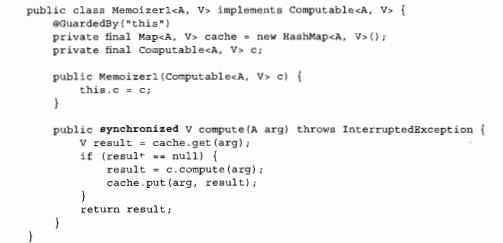class Memoizer<A, V> implements Computable<A, V> {

private final ConcurrentMap<A, Future<V>> cache = new ConcurrentHashMap<A, Future<V>>();

private final Computable<A, V> c;

public Memoizer(Computable<A, V> c) {
this.c = c;
}

@Override
public V compute(final A arg) throws Exception {
while (true) {
Future<V> f = cache.get(arg);
if (f == null) {
Callable<V> callable = new Callable<V>() {
@Override
public V call() throws Exception {
return c.compute(arg);
}
};
f = cache.putIfAbsent(arg, ft);
if (f == null) {
f = ft;
ft.run();
}
}
try {
return f.get();
} catch (CancellationException e) {
cache.remove(arg, f);
} catch (ExecutionException e) {
throw e;
}
}
}
}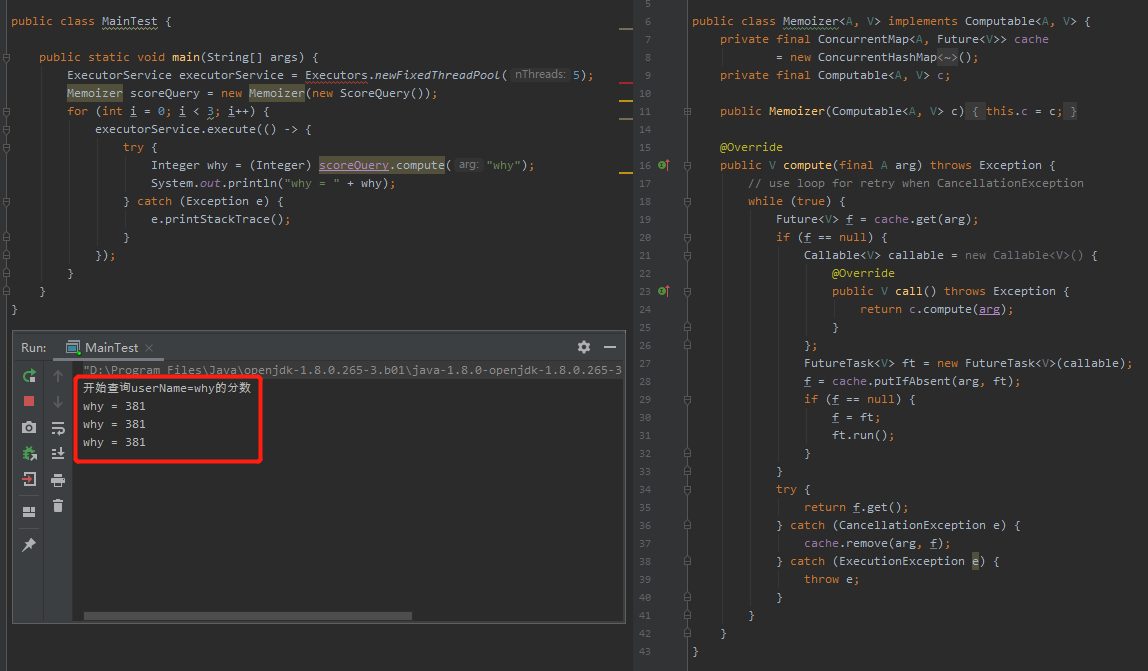Memoizer 给 ScoreQuery 赋能了缓存功能，而且属于润物细无声的那种，完全屏蔽了实现细节。

Memoizer memoizer = new Memoizer(new NucleicAcidTest());《Java并发编程实战》这本书，我都推荐过很多次了，我再推荐一次。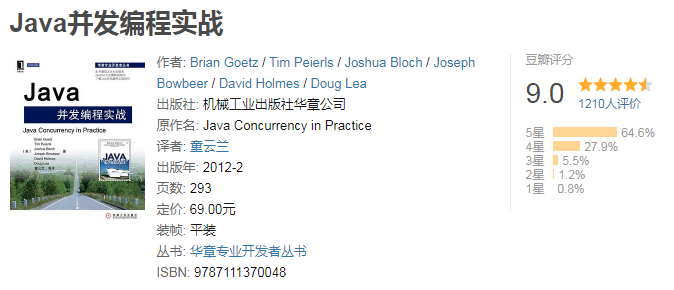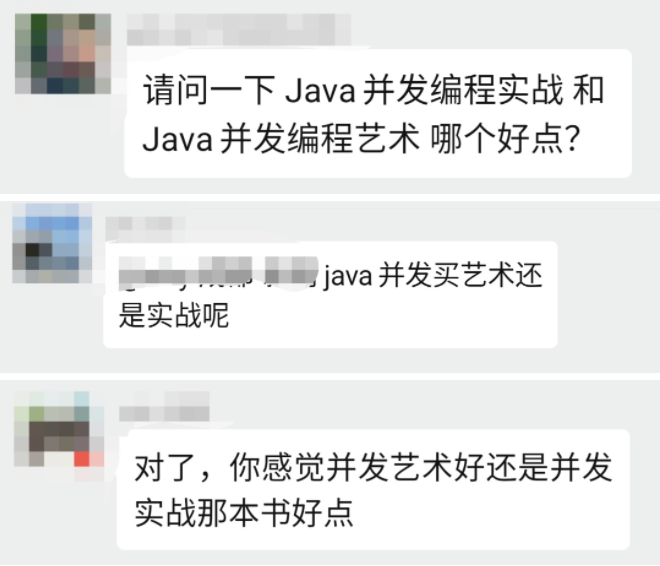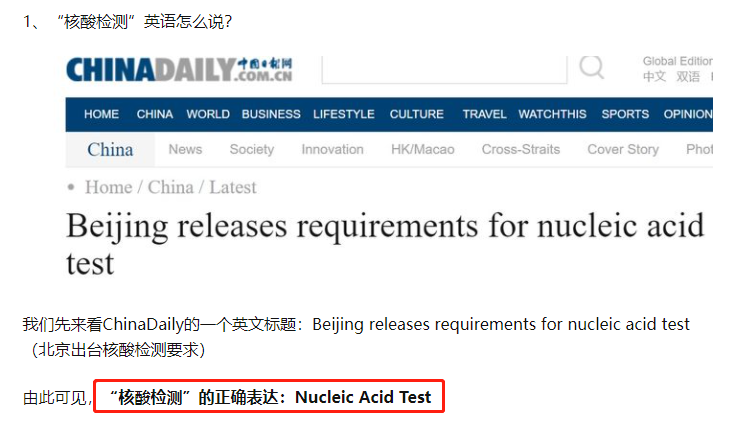## 说个题外话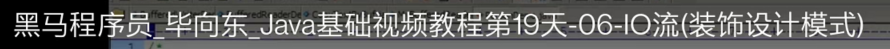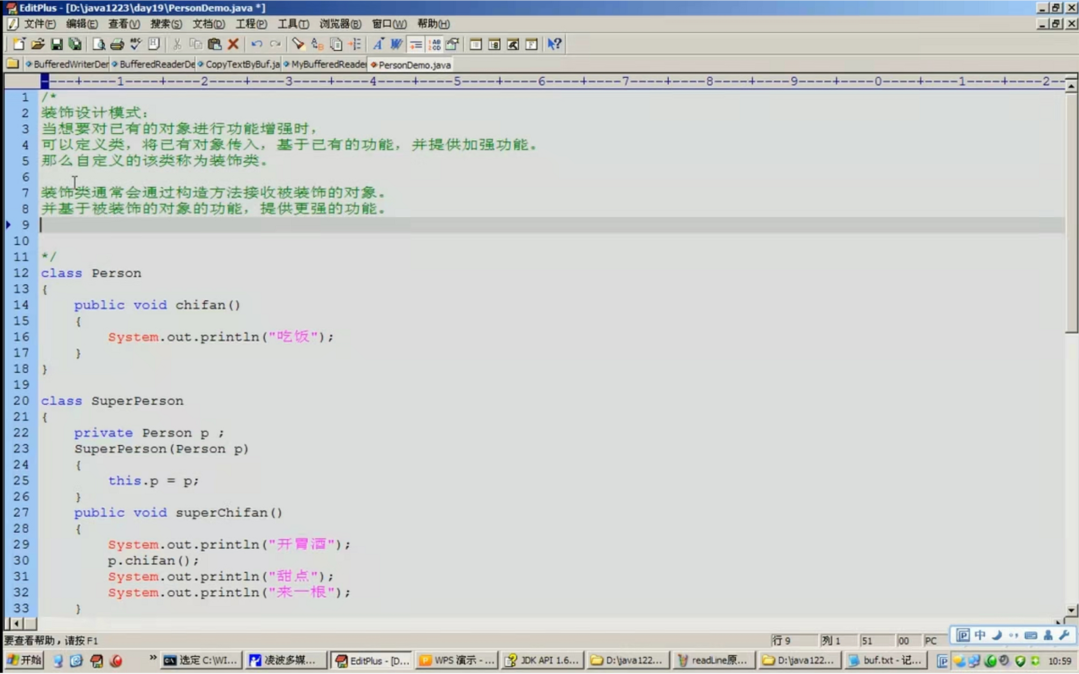## 最后说一句

《构建高效且可伸缩的结果缓存》

《当Synchronized遇到这玩意儿，有个大坑，要注意！》

《几行烂代码，我赔了16万。》

《仔细思考之后，发现只需要赔6w。》

《我就辣鸡怎么了？》0